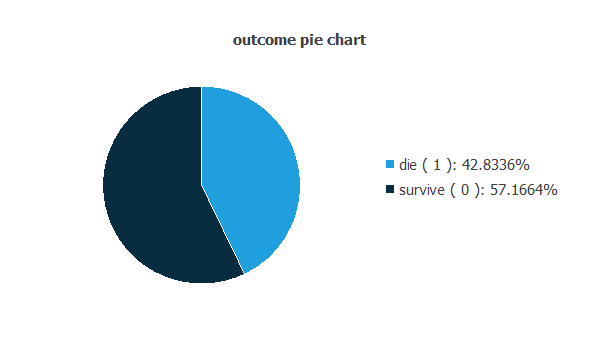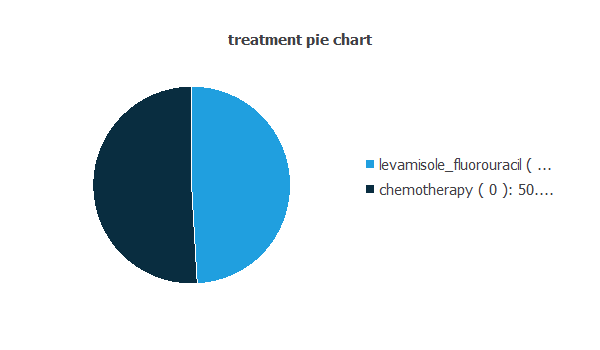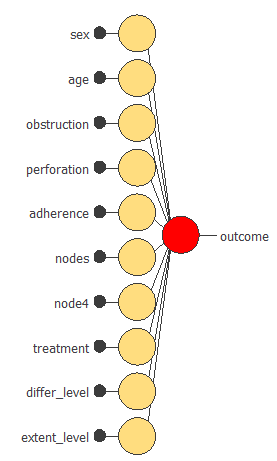# Different treatments after suffering a colon cancer operation using machine learning

This example examines data from a rct, measuring the effect of a particular drug combination on colon cancer. Specifically, we are looking the effect of Levamisole and Fluorouracil on patients who have had surgery to remove their colon cancer.

After surgery, the evolution of the patient depends on the remaining residual cancer. In this study, we see which particular drug combination had a beneficial effect, Chemotherapy or Levamisole and Fluorouracil.### Contents:

This example is solved with Neural Designer. To follow it step by step, you can use the free trial.

## 1. Application type

This is a binary classification project, since the variable to be predicted can have two values (died within 5 years or not).

The goal here is to model the probability whether a patient lives for 5 years or not after being treated with Levamisole and Fluorouracil or Chemotherapy.

## 2. Data set

The coloncancer.csv file contains the data for this application. In a classification project type, target variables can only have two values: 0 (false) or 1 (true). The number of instances (rows) in the data set is 607, and the number of variables (columns) is 15.

The number of input variables, or attributes for each sample, is 12. All input variables are binary or numeric-valued. The number of target variables is 1 and represents whether the patient survive for 5 years or not after being treat. The following list summarizes the variables information:

• sex: (binary). 1 if male, 0 female.
• age: (numeric). Age of patient at start of the study.
• obstruction: (binary). Obstruction of colon by tumor.
• perforation: (binary). Perforation of colon.
• nodes: (numeric). Number of lymphnodes with detectable cancer.
• node4: (binary). More than 4 positive lymph nodes.
• outcome: (binary). 1 if died within 5 years and 0 if not.
• treatment: (binary). 1 if treated with levamisole and fluorocil and 0 with chemotherapy.
• differ_level: (binary, different field for each level). Differentiation of tumor, where level_2 is intermediate forms of tumour with both good and bad prognosis and level_3 is that tumours spread easier than other tumours, and their prognosis is a little worse than for others.
• extent_level: (binary, different field for each level). Extent of local spread. 2 = the cancer has grown into the outermost layers of the colon or rectum but has not gone through them. It has not reached nearby organs. It has not spread to nearby lymph nodes or to distant sites.
• 3 = the cancer has grown through the mucosa into the submucosa. It has spread to 4 to 6 nearby lymph nodes. It has not spread to distant sites. 4 = the cancer may or may not have grown through the wall of the colon or rectum. It might or might not have spread to nearby lymph nodes. It has spread to 1 distant organ (such as the liver or lung) or distant set of lymph nodes, but not to distant parts of the peritoneum (the lining of the abdominal cavity).

Finally, the use of all instances is set. Note that each instance contains the input and target variables of a different patient. The data set is divided into training, validation, and testing subsets. 60% of the instances will be assigned for training, 20% for generalization, and 20% for testing.

Once the data set has been set, we are ready to perform a few related analytics. With that, we check the provided information and make sure that the data has good quality.

We can calculate the data statistics and draw a table with the minimums, maximums, means, and standard deviations of all variables in the data set. The next table depicts the values.Also, we can calculate the distributions for all variables. The following pie chart shows the distribution for the binary variable outcome. The percentage of samples of category die(42.8336%) is greater than the percentage of samples of category survive(57.1664%).Besides, the following pie chart shows the distribution for the binary variable outcome.The percentage of samples of category levamisole_fluorouracil is nearly the same as the percentage of samples of category chemotherapy(50.9061%).

The inputs-targets correlations might indicate to us what factors most influence the target outcome with the input variables of the data set.Here, the most correlated variables are nodes, node4, and extent_level.

## 3. Neural network

The second step is to set a neural network to represent the classification function. For this class of applications, the neural network is composed of:

• Scaling layer.
• Perceptron layers.
• Probabilistic layer.

The scaling layer contains the statistics on the inputs calculated from the data file and the method for scaling the input variables. Here the minimum and maximum method has been set. Nevertheless, the mean and standard deviation method would produce very similar results.

A perceptron layer with a hidden logistic layer is used. Note that, since the logistic function ranges from 0 to 1, the outputs from that layer can be interpreted as probabilities. The neural network must have 13 input variables and 1 target variable. As an initial guess, we use 3 neurons in the hidden layer.

The probabilistic layer only contains the method for interpreting the outputs as probabilities. Indeed, as the sum of all outputs from a probabilistic layer must be 1, that two methods would always yield 1 here since there is only one output. Moreover, as the output layer's activation function is the logistic, that output can already be interpreted as a probability of class membership.

The next figure is a graphical representation of this neural network for colon cancer treatment.It contains a scaling layer, a neural network and a probabilistic layer. The yellow circles represent scaling neurons, the blue circles perceptron neurons and the red circles probabilistic neurons. The number of inputs is 10, and the number of outputs is 1.

## 4. Training strategy

The fourth step is to set the training strategy, which is composed of two terms:

• A loss index.
• An optimization algorithm.

The loss index is the mean squared error with L1 regularization. This is the default loss index for binary classification applications.

The learning problem can be stated as finding a neural network that minimizes the loss index. That is, a neural network that fits the data set (error term) and does not oscillate (regularization term).

The optimization algorithm that we use is the quasi-Newton method. This is also the standard optimization algorithm for this type of problem.

The following chart shows how the error decreases with the iterations during the training process. The final training and selection errors are training error = 0.221 WSE and selection error = 0.231 WSE, respectively.The initial value of the training error is 0.250482, and the final value after 56 epochs is 0.219827. The initial value of the selection error is 0.365811, and the final value after 56 epochs is 0.218302. There is convergence as times increase.

## 5. Model selection

The objective of model selection is to find the network architecture with the best generalization properties, that is, which minimizes the error on the selected instances of the data set.

More specifically, we want to find a neural network with a selection error of less than 0.231 WSE, which is the value that we have achieved so far.

Order selection algorithms train several network architectures with a different number of neurons and select that with the smallest selection error.

The incremental order method starts with a small number of neurons and increases the complexity at each iteration. The following chart shows the training error (blue) and the selection error (orange) as a function of the number of neurons.We appreciate that the error does not change a lot after using model selection, then the neurnos are the same cuantity.

## 6. Testing analysis

The objective of the testing analysis is to validate the generalization performance of the trained neural network. To validate a classification technique, we need to compare the values provided by this technique to the observed values. We can use the ROC curve as it is the standard testing method for binary classification projects.The following table contains the elements of the confusion matrix. This matrix contains the true positives, false positives, false negatives, and true negatives for the variable diagnose.

Predicted positive Predicted negative
Real positive 19(15%) 25(20%)
Real negative 15(12%) 62(51%)

The binary classification tests are parameters for measuring the performance of a classification problem with two classes:

• Classification accuracy (ratio of instances correctly classified): 66.94%
• Error rate (ratio of instances misclassified): 33.05%
• Sensitivity (ratio of real positive which are predicted positive): 72.72%
• Specificity (ratio of real negative which are predicted negative): 57.14%

## 7. Model deployment

Once the neural network's generalization performance has been tested, the neural network can be saved for future use in the so-called model deployment mode.

We can diagnose new patients by calculating the neural network outputs. For that we need to know the input variables for them. An example is following:

• sex: 1
• age: 40
• obstruction: no
• perforation: no
• nodes: 3
• node4: 0
• treatment: chemotherapy
• differ_level: level_2
• extent_level: 2
• diagnose: there is a 40% of probability that the patient will die before 5 years.

We can plot directional outputs to study the behavior of the output variable outcome (died within 5 years or not) as the function of single inputs.The above plot shows the output outcome as a function of the input treatment. The x and y axes are defined by the range of the variables treatment and outcome, respectively. The patient will have more probability to survive if the treatment is Chemotherapy.

In a descriptive level, the amount of patient that survive is 343. Chemotherapy is used in 160 patients and Levamisole and Fluorouracilin 188. As we can appreciate, there is no a huge difference and the data set does not provide a lot of information.

The mathematical expression represented by the neural network is written below. It takes the inputs sex, age, obstruction, perforation, adherence, nodes, node4, treatment, differ_level, extent_level, to produce the output outcome. In classification models, the information is propagated in a feed-forward fashion through the scaling layer, the perceptron layers and the probabilistic layer.

```scaled_sex = (sex-(0.497529))/0.5004060268;
scaled_age = age*(1+1)/(85-(18))-18*(1+1)/(85-18)-1;
scaled_obstruction = (obstruction-(0.1911039948))/0.3934949934;
scaled_perforation = perforation*(1+1)/(1-(0))-0*(1+1)/(1-0)-1;
scaled_nodes = nodes*(1+1)/(27-(0))-0*(1+1)/(27-0)-1;
scaled_node4 = (node4-(0.2685340047))/0.443562001;
scaled_treatment = (treatment-(0.4909389913))/0.5003299713;
scaled_differ_level = (differ_level-(0.7149919868))/0.4517909884;
scaled_extent_level = (extent_level-(2.937289953))/0.3952679932;

perceptron_layer_0_output_0 = sigma[ -0.00253292 + (scaled_sex*-0.000652637)+ (scaled_age*0.00405147)+ (scaled_obstruction*2.60044e-05)+ (scaled_perforation*0.00131418)+ (scaled_adherence*-4.26003e-05)+ (scaled_nodes*2.65084e-05)+ (scaled_node4*-0.213286)+ (scaled_treatment*-5.45851e-05)+ (scaled_differ_level*0.000339709)+ (scaled_extent_level*-0.000982221) ];
perceptron_layer_0_output_1 = sigma[ -0.00367069 + (scaled_sex*5.99516e-05)+ (scaled_age*-0.000703708)+ (scaled_obstruction*0.00100796)+ (scaled_perforation*-0.000147959)+ (scaled_adherence*-5.99783e-05)+ (scaled_nodes*-0.00174084)+ (scaled_node4*9.58423e-05)+ (scaled_treatment*0.00129182)+ (scaled_differ_level*0.00336324)+ (scaled_extent_level*0.000162734) ];

probabilistic_layer_combinations_0 = -0.0027652 -0.311863*perceptron_layer_0_output_0 -0.000413336*perceptron_layer_0_output_1

outcome = 1.0/(1.0 + exp(-probabilistic_layer_combinations_0);

```

The above expression can be exported anywhere, for instance, to a dedicated diagnosis software to be used by doctors.